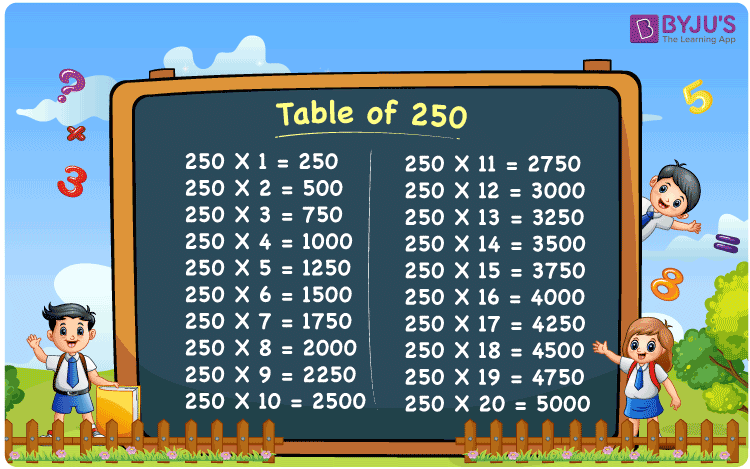Checkout JEE MAINS 2022 Question Paper Analysis : Checkout JEE MAINS 2022 Question Paper Analysis :

# Table of 250

The Table of 250 is provided here up to 20 times. Students can quickly check the multiples of 250 presented here in tabular form. They can download PDF format of the multiplication table of 250 up to 20, which will benefit them learning the 250 times table, and also improve mental math which is an essential skill for any competitive exam.

## Table of 250 Chart

The table of 250 chart is given here to assist students in reviewing the multiples of 250 up to 20 whenever needed. The image of the table of 250 presented can be used as a flashcard while memorising the multiplication table of 250.## What is the 250 Times Table?

The 250 times table lists multiples of 250, which is the multiplication of any natural number with 250. The table of 250 can also be represented as the repeated addition of 250 . The 250 times table is given below both in multiplication and addition form.

 250 × 1 = 250 250 250 × 2 = 500 250 + 250 = 500 250 × 3 = 750 250 + 250 + 250 = 750 250 × 4 = 1000 250 + 250 + 250 + 250 = 1000 250 × 5 = 1250 250 + 250 + 250 + 250 + 250 = 1250 250 × 6 = 1500 250 + 250 + 250 + 250 + 250 + 250 = 1500 250 × 7 = 1750 250 + 250 + 250 + 250 + 250 + 250 + 250 = 1750 250 × 8 = 2000 250 + 250 + 250 + 250 + 250 + 250 + 250 + 250 = 2000 250 × 9 = 2250 250 + 250 + 250 + 250 + 250 + 250 + 250 + 250 + 250 = 2250 250 × 10 = 2500 250 + 250 + 250 + 250 + 250 + 250 + 250 + 250 + 250 + 250 = 2500

## Multiplication Table of 250

Given below is the multiplication table of 250 up to 20.

 250 × 1 = 250 250 × 2 = 500 250 × 3 = 750 250 × 4 = 1000 250 × 5 = 1250 250 × 6 = 1500 250 × 7 = 1750 250 × 8 = 2000 250 × 9 = 2250 250 × 10 = 2500 250 × 11 = 2750 250 × 12 = 3000 250 × 13 = 3250 250 × 14 = 3500 250 × 15 = 3750 250 × 16 = 4000 250 × 17 = 4250 250 × 18 = 4500 250 × 19 = 4750 250 × 20 = 5000

## Solved Examples on the Table of 250

Example 1:

What is the sum of the 17th and the 12th multiple of 250?

Solution:

17th multiple of 250 = 250 × 17 = 4250

12th multiple of 250 = 250 × 12 =  3000

Then, 4250 + 3000 = 7250.

Example 2:

What is 32 times 250?

Solution:

Using the multiplication tables of 250,

250 × 32 = 250 × ( 30 + 2) = (250 × 30) + (250 × 2)

= 7500 + 500 = 8000.

Example 3:

Find the square of 250.

Solution:

Square of 250 = 250 × 250 = 250 × (200 + 50) = 50000 + 12500 = 62500.

## Frequently Asked Questions on Table of 250

### What is the times table of 250?

The times table of 250 is a list of multiples of 250 starting from 250 × 1 = 250, 250 × 2 = 500, 250 × 3 = 750, 250 × 4 = 1000, 250 × 5 = 1250 and so on.

### Which times table has 250?

250 comes in the times tables of 1, 2, 5, 10, 25, 50, 125 and 250.

### What are the factors of 250?

The factors of 250 are 1, 2, 5, 10, 25, 50, 125 and 250. The prime factors of 250 are 2 and 5. The prime factorisation of 250 is 2 × 5 × 5 × 5.

### Is 250 a prime number?

No, 250 is a composite number with factors 1, 2, 5, 10, 25, 50, 125 and 250.

### How many times 250 is 10,000?

40 times 250 is 10,000.